## Example Questions

### Example Question #51 : Arithmetic

Quantity A: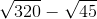Quantity B: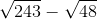Which of the following is true?

Quantity A is larger.

The relationship cannot be determined from the information provided.

Quantity B is larger.

The two quantities are equal.

Quantity A is larger.

Explanation:

Begin by breaking down each of the roots in question.  Often, for the GRE, your answer arises out of doing such basic "simplification moves".

Quantity AThis is the same as: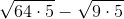, which can be reduced to: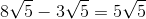Quantity BThis is the same as: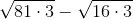, which can be reduced to: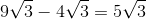Now, since we know that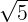must be greater than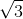, we know that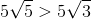.  Therefore, quantity A is larger.

### Example Question #52 : Arithmetic

Solve for.

Note,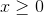: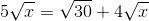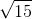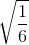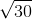Explanation:

Begin by subtracting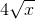from both sides: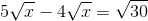This simplifies to: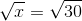Now, while you could square both sides, you also know thatmerely needs to be the same as the value under the other radical. This means that it is—simply and quickly! (Always work to save time on the exam!)

### Example Question #53 : Arithmetic

Quantity A: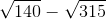Quantity B: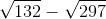Which of the following is true?

The relationship cannot be determined from the information provided.

Quantity B is larger.

The two quantities are equal.

Quantity A is larger.

Quantity B is larger.

Explanation:

As always for comparison questions, work on getting your quantities explicit. In order to do this in this case, you must factor the roots.

Quantity A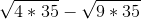This can be factored into: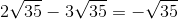Quantity B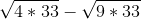This can be factored into: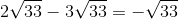This makes the comparison very easy! Sinceis a larger number than, we know that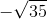is a smaller value than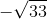. Therefore, Quantity B is larger.

Tired of practice problems?

Try live online GRE prep today.